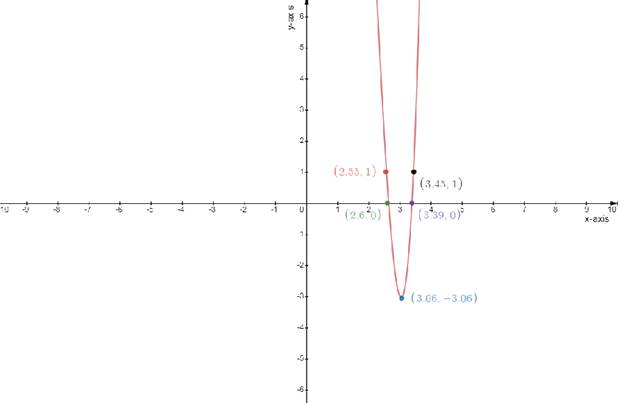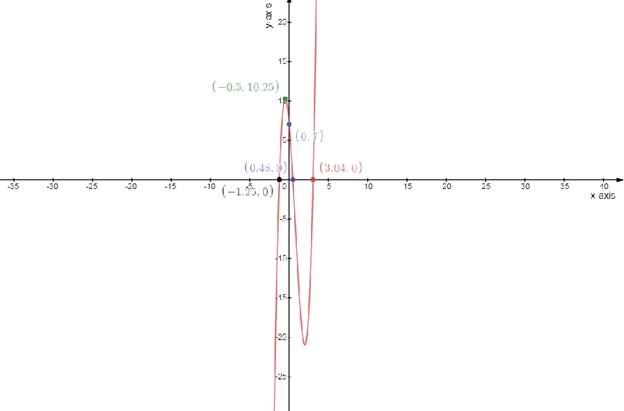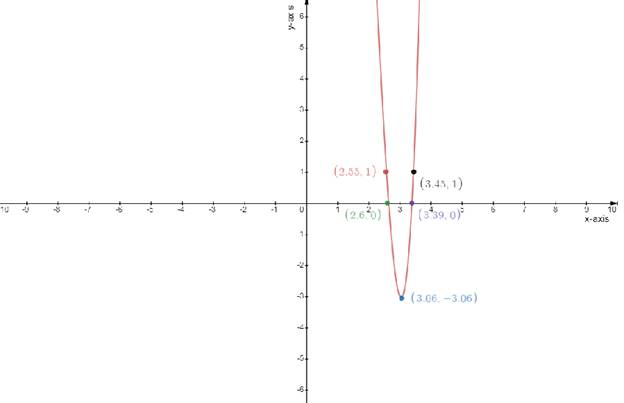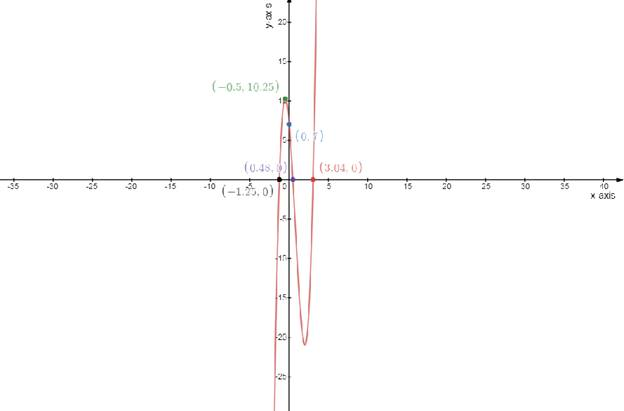# To draw: The graph of the given function### Single Variable Calculus: Concepts...

4th Edition
James Stewart
Publisher: Cengage Learning
ISBN: 9781337687805### Single Variable Calculus: Concepts...

4th Edition
James Stewart
Publisher: Cengage Learning
ISBN: 9781337687805

#### Solutions

Chapter 3.1, Problem 39E

(A)

To determine

## To draw:The graph of the given function

Expert Solution

### Explanation of Solution

Given:

The function is

f(x)=x43x36x2+7x+30

Concept used:

Definition of the differentiation:-Differentiation is the action of computing a derivative

The derivative of a function y=f(x) of a variable x is a measure of the rate at which the value y of the function changes with respect to x

Calculation:

The function

f(x)=x43x36x2+7x+30...................(1)

Draw the table

f(x)=x43x36x2+7x+30

Test one point in each of the region formed by the graph

If the point satisfies the function then shade the entire region to denote that every point in the region satisfies the function

 x−axis 3.06 3.39 3.45 2.55 y−axis −3.06 0 1 1(B)

To determine

Expert Solution

### Explanation of Solution

Given:

The function is

f(x)=x43x36x2+7x+30

Concept used:

Definition of the differentiation:-Differentiation is the action of computing a derivative

The derivative of a function y=f(x) of a variable x is a measure of the rate at which the value y of the function changes with respect to x

Calculation:

The function

f(x)=x43x36x2+7x+30f(x)=4x39x212x+7...................(1)

Draw the table

f(x)=4x39x212x+7

Test one point in each of the region formed by the graph

If the point satisfies the function then shade the entire region to denote that every point in the region satisfies the function

 x−axis 0 −0.5 0.48 −1.25 y−axis 7 10.25 0 0(C)

To determine

Expert Solution

### Explanation of Solution

Given:

The function is

f(x)=x43x36x2+7x+30

Concept used:

Definition of the differentiation:-Differentiation is the action of computing a derivative

The derivative of a function y=f(x) of a variable x is a measure of the rate at which the value y of the function changes with respect to x

Calculation:

The function

f(x)=x43x36x2+7x+30...................(1)

Draw the table

f(x)=x43x36x2+7x+30

Test one point in each of the region formed by the graph

If the point satisfies the function then shade the entire region to denote that every point in the region satisfies the function

 x−axis 3.06 3.39 3.45 2.55 y−axis −3.06 0 1 1Draw the table

f(x)=4x39x212x+7

Test one point in each of the region formed by the graph

If the point satisfies the function then shade the entire region to denote that every point in the region satisfies the function

 x−axis 0 −0.5 0.48 −1.25 y−axis 7 10.25 0 0### Have a homework question?

Subscribe to bartleby learn! Ask subject matter experts 30 homework questions each month. Plus, you’ll have access to millions of step-by-step textbook answers!